# Introduction to emojifont package in R

• Last Updated : 26 Apr, 2022

In this article, we will see how to use emojifont package in R. This package is designed to bring emoji font to R Programming Language.

We will have a look at how to search and print emoji using emojifont package.

## Installation:

To install this package type the below command in the terminal.

install.packages(“emojifont”)

## Emoji characters in R

Now we have emojifont package installed so we will import it using library(emojifont). To print emoji we will use emoji(“emojiname”) to search emoji we will use search_emoji(“name”), this will return all the related emoji and we will store it as a list and print using emoji().

## R

 `# importing package` `library``(emojifont)`   `# printing joy emoji` `emoji``(``"joy"``)`   `# searching emoji` `list <- ``c``(``search_emoji``(``"joy"``))`   `# printing all the emoji's in the list` `emoji``(list)`

Output: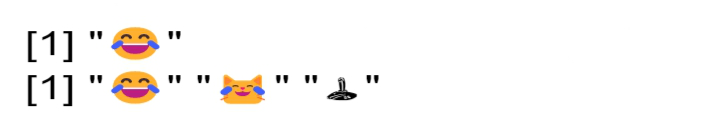Emoji characters in R

## Getting a random sample of emoji

To get random emoji we will be using sample_emoji(x) to get random emoji’s.

## R

 `# importing package` `library``(emojifont)`   `# getting random sample of emojis` `list = ``c``(``sample_emoji``(10))`   `# printing all the emoji's in list` `emoji``(list)`

Output: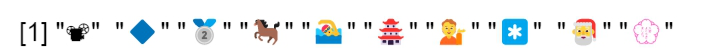random sample of emoji

## Emoji in R plot

### Method 1: Using Base Plot

plot() is used to plot the chart where x and y are coordinates.

plot(x, y, …)

“…” are the arguments passed to methods like graphicals parameters.

text() is used to draw vectors labels. where x,y coordinates,

text (x, y = NULL, labels = seq_along(x\$x), cex = 1, col = NULL, …)

## R

 `# importing package` `library``(emojifont)`     `# generating random numbers for x-axis` `set.seed``(200)`   `# gets 10 random point on x axis from ` `# seed` `x <- ``rnorm``(10)`   `# generating random numbers for y-axis` `set.seed``(100)`   `# gets 10 random point on y axis from ` `# seed` `y <- ``rnorm``(10)`   `# Plotting a blank chart in order to ` `# clear the screen` `# if any chart is already opened` `# or the emoji's will overlap` `plot``(x, y, cex = 0)`   `# plotting chart` `text``(x, y, labels=``emoji``(``'joy'``), cex=3.5, col=``'red'``,` `     ``family=``'EmojiOne'``)`

Output: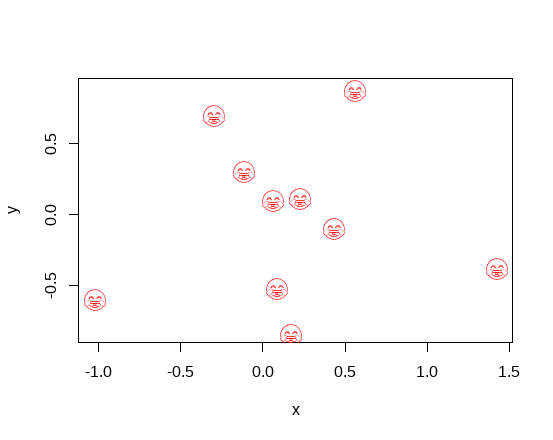### Method 2: Using ggplot

ggplot() is used to plot chart, where data is our data frame, aes() describes how content will be plotted on the screen.

ggplot(data = NULL, mapping = aes(), …)

## R

 `# importing package` `library``(emojifont)` `library``(ggplot2)`   `# generating random numbers for x-axis` `set.seed``(200)`   `# gets 10 random point on x axis from` `# seed` `x <- ``rnorm``(10)`   `# generating random numbers for y-axis` `set.seed``(100)`   `# gets 10 random point on y axis from` `# seed` `y <- ``rnorm``(10)`   `# defining data frame` `data <- ``data.frame``(x=x,y=y)`   `# plotting chart` `ggplot``(data,``aes``(x, y, color=``"red"``, label=``emoji``(``'joy'``))) +` `  ``geom_text``(family=``"EmojiOne"``, size=6)`

Output: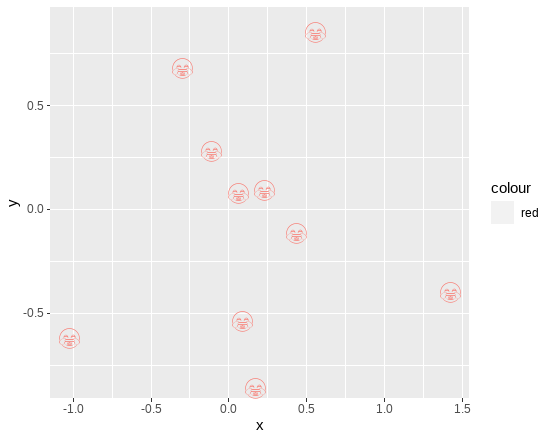### Method 3: Using Geom_emoji

geom_emoji() works as a wrapper for visualizing emoji.

## R

 `# importing package` `library``(emojifont)` `library``(ggplot2)`   `# plotting emoji` `ggplot``() + ``geom_emoji``(``"joy"``, color=``'red'``) + ``theme_void``()`

Output: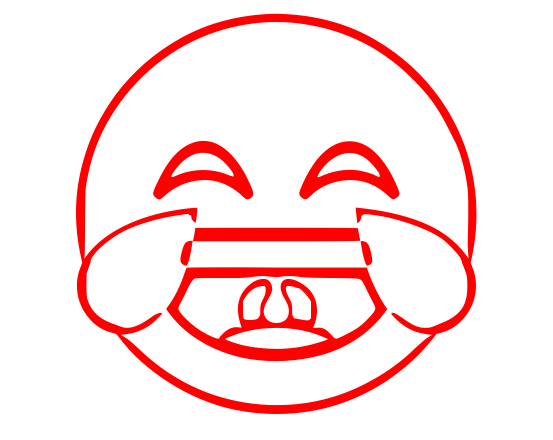### Method 4: Font Awesome

geom_fontawesome() works as a wrapper for visualizing fontawesome.

## R

 `# importing package` `library``(emojifont)` `library``(ggplot2)`   `# plotting emoji` `ggplot``() + ``geom_fontawesome``(``"fa-desktop"``, ` `                            ``color=``'black'``) + ``theme_void``()`

Output:My Personal Notes arrow_drop_up
Recommended Articles
Page :